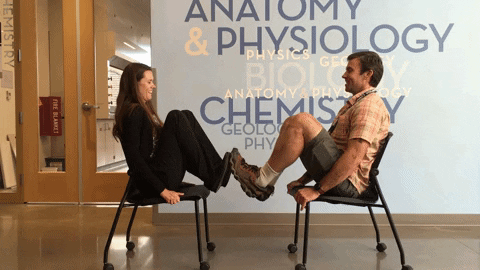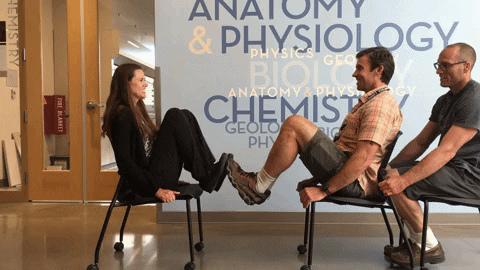# Collisions

So far we have analyzed forces applied to the body by the earth during impact after a fall and during locomotion such as walking, jumping, and landing. These events are examples of collisions. Even though we usually use the word collision to refer to objects that were initially separated and came together, in fact any event that involves objects applying force on one another can be considered a collision and analyzed a such. In each of the collision we have analyzed so far we assumed one of the objects (the earth) did not change velocity when it applied force to the body, even though we know from Newton’s Third Law that earth felt a force back from the body. This was a reasonable assumption because the earth is so much larger than a human body, but ignoring the motion of the larger object in a collision is not always reasonable.  For example, the following GIF shows a basic form of locomotion in which two objects push against one another and separate.Sitting in a wheeled chairs, Science Lab Technician Becky Kipperman (left) and Associate Chemistry Professor Sean Breslin (right) push against one another. This collision causes a leftward change in motion for Becky and a smaller rightward change in motion for Sean.

Notice that both objects experienced a noticeable change in velocity, but the velocity changes were not equal in size.  In this example, the wheels minimize friction so that the net horizontal force on each person is just the force from the other person. Newton’s Third Law tells us that these forces are equal in size, so why does one object experience a greater change in velocity, even though they have the same net force? Newton’s Second Law provides the answer: given the same net force, a more massive object will experience a smaller acceleration.

(1)This effect becomes more apparent when the difference in mass between the objects is greater:Sitting in a wheeled chairs, Science Lab Technician Becky Kipperman (left) and Associate Chemistry Professor Sean Breslin (right) push against one another while the author is attached to Sean in another chair, but not touching the floor. This collision causes a leftward change in motion for Becky and a much smaller rightward change in motion for Sean and the author. The objects experience equal and opposite changes in momentum though.

The combined mass of Sean and the author is more than 3x the mass of Becky, therefore they experience only 1/3 the change in velocity. This mode of locomotion is really no different from you pushing against the ground when walking. When you push off the ground to walk forward you cause the Earth to move back in return. When you do a push-up, you actually push the Earth down just a little bit. Go you!

# Momentum

So far we have been ignoring the change in motion of the earth because the mass of the earth is so large that its change in motion is. For example, when analyzing impact forces we assumed that the final velocity of the body was zero after a collision with the earth and we used that final velocity to calculate acceleration and then net force. However, when objects of similar mass collide we have to keep track of all objects in the collision, which we call the system, because the final velocity of the objects in the system after the collision might not be zero. For example, in this video of a car accident we see that both cars are moving after the collision (they eventually come to rest due to friction, but we can analyze that separately from the collision forces).

To analyze how mass affects changes in velocity during a collision we use the combination of times (), which is known as the (). Written in symbol form, the momentum is:

(2)# Conservation of Momentum

The states that the combined total momentum of all objects in a system must be the same immediately before and immediately after a collision:

(3)If we have only two objects and their masses do not change during the collision we can write the Law of Conservation of Momentum as:

(4)### Everyday Example: Locomotion

When analyzing locomotion we have ignored the motion of the earth. Let’s verify that to be a reasonable assumption. A person of about 65 kg mass starts from rest and takes a step so they have a velocity of 1.2 m/s at the end of the step. What is the resulting velocity of the earth? We only have two objects of constant mass (body and earth) so we will use the previous equation:The initial velocity of both objects is zero, (so their initial momenta are also zero):Now we subtract the initial momentum of the person (object #2) to the left side:Finally we divide by the mass of the earth to isolate the final velocity of the earth:And switching sides to have the final earth velocity on the left we are ready to enter numbers. We just need to look up the mass of the earth:The mass of the earth isso we have:That’s a final earth velocity of 14 with 22 zeros in front of it, or about the width of an atom per million years. I think we were justified in neglecting the earth velocity in our analysis so far.

### Everyday Examples: Collisions

Let’s analyze the car accident in the previous video. The initial momentum of the stopped car (car #1) was zero:.  The momentum of the jeep (car #2) was:. Therefore the total initial momentum wasThe cars lock together immediately after the collision and only separate later so they have the same final velocity immediately after the collision, we’ll call it. Sticky collisions like this are known as , and for such collisions we can treat the objects moving together as a single object that has their combined total . The final is then:Conserving momentum during the collision tells us to set the initial and final momenta equal:If we want to solve this equation for the final velocity we divide by the combined mass:We can look up some data on the cars and find that the length of the Jeep is 3.8 m,  the mass of the jeep is about 1500 kg and a small sports car mass is about 1000 kg Now let’s estimate some numbers from the video (a quick method is to use the slo-mo feature on a smartphone to film the video as it plays with a running stopwatch also visible in the frame).  We see that before the collision the Jeep covers at least two of its own lengths in about 0.4 s so calling its direction the positive, its initial velocity will be:We are ready to calculate the final velocity of the jeep-car combination:This is an interesting result, but what’s really cool is that if we estimate the collision interval we can calculate the average force applied to each car. From the video the collision time appears to be about 0.5 s. Let’s do the Jeep first.

We start from Newton’s Second Law:

(5)Then write out the acceleration as change in velocity over change in time:

(6)We have the final velocity of 12 m/s from our previous work using momentum conservation, so we can enter all our values:

(7)The force on the jeep should be in the negative, just as we found, because  we originally chose  backward as the negative direction.We known from that the -23,000 N force on the Jeep from the car is paired with a 23,000 force felt by the car from the Jeep. That’s over 5,000 lbs of average force on each vehicle, the peak force would be much greater.

### Reinforcement Exercise